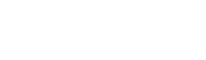# 【OpenCV・Numpy】Calculate the L1-norm mean and L1-norm variance of RGB images

スポンサーリンク

Hello, I’m Yuki (@engieerblog_Yu), a data science student in the Visualization Laboratory.

This time, I had a chance to obtain the L1-norm mean and L1-norm variance of an image for my research, so I would like to briefly explain about them.

スポンサーリンク

## What are norm mean and norm variance?

The norm mean and norm variance are statistics that describe the change in luminance values and colors in an image.

・Norm mean (L1 mean): The sum of the values of all pixels in an image added together and the sum divided by the number of pixels in the image.
・Norm Variance (L1 Variance): The sum of the squares of the differences between the values of all pixels in the image and the norm mean, divided by the number of pixels in the image.

## Coding

import cv2
import numpy as np

# 画像の読み込み

# ノルム平均を求める
norm_mean = np.sum(img) / img.size

# ノルム分散を求める
norm_var = np.sum((img - norm_mean) ** 2) / img.size

The number of pixels in the image is obtained in img.size.

In addition, the image is read in RGB, so it contains the values of the three channels, R, G, and B.

Therefore, the above code adds up the values of all three channels, R, G, and B.

## At the End

In this article, we introduced Python code to find the norm mean and norm variance of an image.